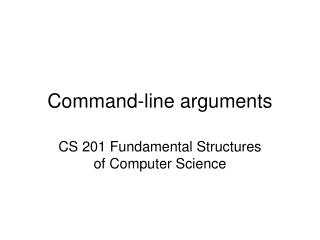DownloadDownload PresentationCommand-line arguments

# Command-line arguments

Download Presentation## Command-line arguments

- - - - - - - - - - - - - - - - - - - - - - - - - - - E N D - - - - - - - - - - - - - - - - - - - - - - - - - - -
##### Presentation Transcript

1. Command-line arguments CS 201 Fundamental Structures of Computer Science

2. Introduction • A command-line argument is the information that follows the name of the program on the command line of the operating system • Command-line arguments are used to pass information into a program when you run it • They facilitate the use of your program in batch files • They give a professional appearance to your program

3. Introduction • C++ defines two built-in parameters to main() • They receive the command line arguments • Their names are argc and argv • The names of the parameters are arbitrary. However, argc and argv have been used by convention for several years. • They are optional

4. int main(int argc, char *argv[]) • argc is an integer • Holds the number of arguments on the command line • Since the name of the program always corresponds to the first argument, it is always at least 1

5. int main(int argc, char *argv[]) • argv is a pointer to an array of character pointers. • Each character pointer in the argv array corresponds a string containing a command-line argument • argv points the name of the program, argv points to the first argument, argv points to the second argument, … • Each command-line argument is a string • If you want to pass numerical information to your program, your program should convert the corresponding argument into its numerical equivalent • Each command-line argument must be separated by spaces or tabs • Commas, semicolons, and the like are not valid argument separators

6. #include <iostream> using namespace std; int main(int argc, char *argv[]){ cout <<"Hello "; for (int i = 1; i < argc; i++) cout << argv[i] <<" "; cout << "! " << endl; return 0; } [cgunduz@knuth cgunduz]\$ g++ prog1.cpp –o exe_1 [cgunduz@knuth cgunduz]\$ ./exe_1Cigdem Gunduz Hello Cigdem Gunduz ! [cgunduz@knuth cgunduz]\$ ./exe_1Cigdem Gunduz Demir Hello Cigdem Gunduz Demir !

7. Passing numeric command-line arguments • All command-line arguments are passed to the program as strings • Your program should convert them into their proper internal format • For that, C++ supports standard library functions most commonly used ones are: • atof() : converts a string to a double and returns the result • atoi() : converts a string to a int and returns the result • atol(): converts a string to a long int and returns the result • Each of these functions • Expects a string containing a numeric value as an argument • Uses the header <cstdlib>

8. #include <iostream> #include<cstdlib> using namespace std; int main(int argc, char *argv[]){ if (argc != 4){ cout << "Usage: \n\t"; cout << "1. Integer (0) or double (1) division\n\t"; cout << "2. Operand 1\n\t3. Operand 2\n\n"; exit(1); } if (atoi(argv) == 0){ int a = atoi(argv); int b = atoi(argv); cout << a << "\\" << b << " = " << a / b << endl; } else{ double a = atof(argv); double b = atof(argv); cout << a << "\\" << b << " = " << a / b << endl; } return 0; }

9. [cgunduz@knuth cgunduz]\$ g++ prog2.cpp -o exe_2 [cgunduz@knuth cgunduz]\$ ./exe_2 Usage: 1. Integer (0) or double (1) division 2. Operand 1 3. Operand 2 [cgunduz@knuth cgunduz]\$ ./exe_2 0 5 3 5\3 = 1 [cgunduz@knuth cgunduz]\$ ./exe_2 1 5 3 5\3 = 1.66667 [cgunduz@knuth cgunduz]\$ ./exe_2 0 8.2 2.9 8\2 = 4 [cgunduz@knuth cgunduz]\$ ./exe_2 1 8.2 2.9 8.2\2.9 = 2.82759